# HC Verma Solutions Class 12 Chapter 4 Laws Of Thermodynamics

HC Verma Solutions Class 12 Chapter 4 Laws of Thermodynamics given here will help students to go through all the exercises and improve their ability to tackle all the questions related to the chapter. This is an important unit from an examination point of view. The chapter also focuses on enabling students to learn about fundamental physical properties like entropy, temperature, and energy that are all defined by the laws of thermodynamics. These topics are mainly found under thermal equilibrium.

Having HC Verma Solutions Part 2 will come in handy as it will improve the students’ ability to solve complex questions based on the four laws of thermodynamics: The zeroth law, the First Law, the Second Law, and the Third Law. The solution also contains answers to all the exercises given in the chapter and will ultimately help students to study productively and prepare well for the exams.

The HC Verma solutions are available in a PDF format which students can download and use it to study offline.

## Laws of Thermodynamics Key Topics

The chapter covers some of the topics like;

• Laws of Thermodynamics
• Work Done by Gas
• Heat Engines
• Reversible and Irreversible Processes
• Carnot engine

## Class 12 Important Questions In Chapter 4

1. We apply a certain force F on a block of mass M and is displaced for distance d in the direction of the force. Explain the amount of work done by the force on the block? Also, clarify whether the internal energy changes because of this work or not?

2. State the condition for the efficiency of a Carnot engine to be equal to 1?

3. What does the first law of thermodynamics signify?

(a) conservation of momentum (b) conservation of energy (c) conservation of heat (d) conservation of work

4. Suppose the internal energy of an ideal gas is decreased by the same amount as the work done by the system. What is the process involved?

(a) An adiabatic process (b) The process is isobaric (c) The process must be isothermal (d) There is a decrease in temperature

5. Usually, when a hot object cools down, the heat dissipates from it. In such a process, will the entropy of the object decrease? State whether this process violates the second law of thermodynamics or not?

## HC Verma Solutions Vol 2 Laws Of Thermodynamics Chapter 4

Question 1: A thermally insulated closed copper vessel contains water at 15°C. When the vessel is shaken vigorously for 15 minutes, the temperature rises to 17°C. The mass of the vessel is 100 g and that of the water is 200 g. The specific heat capacities of copper and water are 420 J kg–1 K–1 and 4200 J kg–1 K–1 respectively.

Neglect any thermal expansion.

(a) How much heat is transferred to the liquid-vessel system?

(b) How much work has been done on this system?

(c) How much is the increase in internal energy of the system?

Solution:

Let T1 and T2 be Initial and Final temperature of water respectively.

mc and mw be Mass of copper vessel and water respectively.

cc and cw be Specific heat capacity of copper and water respectively.

Given:

T1 = 15℃ = 288K ; T2 = 17℃ = 290K

mc = 100g = 100×10-3kg ; mw = 200g = 200×10-3kg

cc = 420 J kg–1 K–1 ; cw = 4200 J kg–1 K–1

(a) No heat from the surroundings can be transferred to the liquid-vessel system, as copper vessel is thermally insulated.

b) Work done on this system:

Using formula, ΔW = mw cw ΔT + mc cc ΔT

Temp change = ΔT = T2 – T1 = 290-288 = 2K

=> ΔW = 200 × 10-3 × 4200 × 2 + 100 × 10-3 × 420 × 2 = 1764 J

c)

We know, ΔQ = ΔU + ΔW …(1)

[first law of thermodynamics]

Where ΔQ = heat supplied to the system, ΔU = change in internal energy and ΔW = work done by the system

From part (a), we have ΔQ = 0

(1)=>ΔU = -ΔW

From part (2), ΔW = 1764 J

So, work done by the system ΔW = -1764J

=> ΔU = -(-1764) = 1764J

Increase in internal energy of the system is 1764J.

Question 2: Figure shows a paddle wheel coupled to a mass of 12 kg through fixed frictionless pulleys. The paddle is immersed in a liquid of heat capacity 4200 JK–1 kept in an adiabatic container. Consider a time interval in which the 12 kg block falls slowly through 70 cm.

(a) How much heat is given to the liquid?

(b) How much work is done on the liquid?

(c) Calculate the rise in the temperature of the liquid neglecting the heat capacity of the container and the paddle.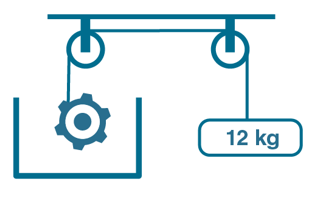Solution:

(a) Heat given to liquid is zero.

As, Paddle immersed in liquid is kept in an adiabatic container. So, no heat can be either supplied or extracted to the liquid.

(b) Work done on the liquid = potential energy of mass

[As, no heat is supplied to liquid and pulley is frictionless]

We know, Potential energy of mass = mgh

Where, m = Mass attaches to the pulley = 12kg (Given)

and h = Height through which mass fall = 70cm = 0.7m (Given)

g = acceleration due to gravity = 10 m/s2

Potential energy of mass = 12×10×0.7 = 84J

=> Work done on the liquid = 84 J

(c) We know, s = ΔQ/ΔT

Where ΔQ = heat supplied and ΔT = rise in temperature

ΔT = ΔQ/s

s = Heat capacity of liquids = 4200 JK-1

Since work done is equal to heat supplied, therefore

ΔT = 84/4200 = 0.02 K

Thus, rise in temperature of the liquid will be 0.02K.

Question 3: A 100 kg block is started with a speed of 2.0 m

s–1 on a long, rough belt kept fixed in a horizontal position. The coefficient of kinetic friction between the block and the belt is 0.20.

(a) Calculate the change in the internal energy of the block-belt system as the block comes to a stop on the belt.

(b) Consider the situation com a frame of reference moving at 2.0 m s–1 along the initial velocity of the block. As seen from this frame, the block is gently put on a moving belt and in due time the block starts moving with the belt at 2.0 ms–1. Calculate the increase in the kinetic energy of the block as it stops slipping past the belt.

(c) Find the work done in this frame by the external force holding the belt.

Solution:

(a) Since the block comes to stop on the belt, final velocity will be zero, i.e. V = 0 m/s

we know that heat is produced due to friction between two surfaces. Now because of this heat, the internal energy of block will change.

Kinetic energy lost = initial kinetic energy- final kinetic energy

= (1/2) mu2 – (1/2) mv2

Here m = 100 kg and u (initial velocity) = 2 m/s and v (final velocity) = 0 m/s [given]

Kinetic energy lost = 200 J

In this frame of reference initial and final velocity of the block will change.

velocity of the frame of reference = uo = 2m/s (Given)

New initial velocity = u’= u – uo = 2-2 = 0 m/s

New final velocity v’ = v-uo = 0 – 2 = -2 m/s

Increase in kinetic energy = Final kinetic energy – Initial kinetic energy

= (1/2) mv’2 – (1/2) mu’2

= (1/2) x 100 x (-2) 2 – 0

= 200 J

(c) work done in this frame by the external force holding the belt.

We know, f = μN

Where, μ = coefficient of friction =0 .02 and

N = normal reaction = mg

=> f = 0.02 x 100 x 10 = 200N

Also, force= mass × acceleration [newton’s second law of motion]

=> 200 = 100 x acceleration

=> acceleration = 2 m/s2

Again, by third equation of motion, we have

v’2 – u’2 = 2as

=> (-2) 2 – 0 = 2x2xs

=> s = 1 m

Work done due to friction Wf = Force × Displacement

Wf = 200 × 1 = 200 J

According to work-energy theorem, Work done = change in kinetic energy

W’ = (1/2)mv’2 – (1/2)mu’2

= (1/2) x 100 x (-2) 2 – 0

= 200 J

Therefore, Total work done = work done in com frame of reference = W = W’ + Wf = 200 + 200 = 400J.

Question 4: Calculate the change in internal energy of a gas kept in a rigid container when 100 J of heat is supplied to it.

Solution:

Given: ΔQ = 100J

From first law of thermodynamics, ΔQ = ΔU + ΔW …(1)

Work done by the gas, ΔW = P ΔV

Since the gas is kept in a rigid container, so ΔV = 0

=> ΔW = 0

(1)=> ΔQ = ΔU = 100 J

change in internal energy will be 100 J.

Question 5: The pressure of gas changes linearly with volume from 10 kPa, 200 cc to 50 kPa, 50 cc.

(a) Calculate the work done by the gas.

(b) If no heat is supplied or extracted from the gas, what is the change in the internal energy of the gas?

Solution:

Given:

P1 = 10 k Pa = 10 × 103 Pa ; P2 = 50 kPa = 50 × 103 Pa

V1 = 200cc = 200 × 10-6 m3 ; V2 = 50cc = 50 × 10-6 m3

Where P1 and P2 be initial and final pressure

v1 and v2 be the Initial and final volume

(a) work done by the gas = ΔW = P ΔV

As, two values of pressure. So, we will take the average value of pressure

P = [P1 + P2]/2 = (1/2)[10 + 50) x 103 = 30 x 103 Pa

ΔV = V2-V1 = (50 -200) x 10-6

work done on the gas = 30 x 103 x (50 -200) x 10-6 = -4.5 J

(b) From first law of thermodynamics, ΔQ = ΔU + ΔW

Since ΔQ=0

Therefore ΔU = -ΔW = -(-4.5) J = 4.5J

Question 6: An ideal gas is taken from an initial state i to a final state f in such a way that the ratio of the pressure to the absolute temperature remains constant. What will be the work done by gas?

Solution:

P1/T1 = P2/T2 (Given)

Where P1 = initial pressure and P2 = final pressure

T1 and T2 are absolute temp.,

Applying ideal gas equation for both processes,(PV = nRT) we get

nR/V1 = nR/V2

=> V1 = V2 ….(1)

We know, ΔW = PΔV

change in the volume of system = ΔV = V2 – V1 = 0 [Using (1)]

=> ΔW = 0

work done by the gas is zero.

Question 7: Figure shows three paths through which a gas can be taken from the state A to the state B. Calculate the work done by the gas in each of the three paths.

Solution: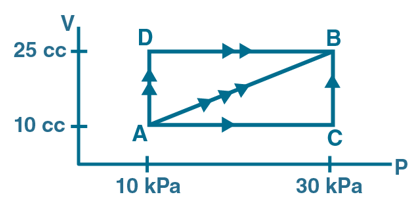We know that work done by the gas: ΔW = PΔV

WADB = PA (VD-VA) + 0

[Because VD-VB, so WDB =0)

From graph,

= 10 × 103 × (25 – 10) × 10-6

= 0.15J

Work done in path AB:

WAB = Pavg(VB – VA)

Here, Pavg = [PB + PA]/2 = [10×103 + 30×103]/2 = 20 x 103 Pa

=> WAB = 20 x 103 (25 – 10)10-6 = 0.30 J

Work done in path ACB:

WACB = WAC + WBC

Here WAC = 0 because VA=VC

WACB = 0 + PB(VB-VC)

= 30×103×(25-10)×10-6

=0.45J

Question 8: When a system is taken through the process abc shown in figure shown below of heat is absorbed by the system and 30 J of work is done by it. If the system does 10 J of work during the process adc, how much heat flows into it during the process?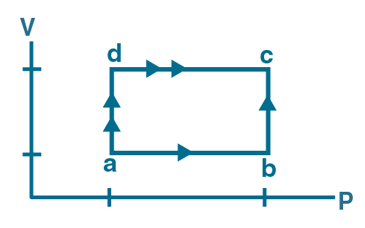Solution:

Given: ΔQ1 = 80J ; ΔW1 =30J ; ΔW2 =10J

Let heat absorbed into the system during process adc = ΔQ2

Using first law of thermodynamics for process abc

ΔQ1 = ΔU1+ΔW1 and ΔQ2=ΔU2+ΔW2

ΔU1 = ΔQ1 – ΔW1 = 80 – 30 = 50J

and

ΔQ2=ΔU2+ΔW2 = ΔU1 + ΔW2 = 50 + 10 = 60 J

Question 9: 50 cal of heat should be supplied to take a system from the state A to the state B through the path ACB as shown in the figure. Find the quantity of heat to be supplied to take it from A to B via ADB.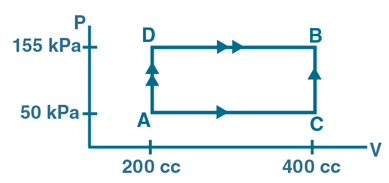Solution:

Heat absorbed in process ABC = ΔQ1 = 50cal = 50×4.2 J = 210J

Let heat absorbed into the system during process ADC = ΔQ2

We know that work done by the gas: ΔW = PΔV

Work done in path ACB:

WACB = ΔW1 = WAC + WBC

=>ΔW1 = PA(VC – VA) + 0

(In figure, VB = VC => WBC = 0 )

= 50 ×103×(400-200)×10-6

= 10J

ΔW2 = PB (VD-VB) + 0

(In figure, VA = VD => WAD=0)

=155×103×(400-200)×10-6

=31 J

From first law of thermodynamics,

For process ABC

ΔQ1 = ΔU1 + ΔW1

Or ΔU1 = 2 10 – 10 = 200J

ΔQ2 = ΔU2 + ΔW2

= ΔU1 + ΔW2

[ΔU1=ΔU2=ΔU as, initial point and final point for both the processes is the same. So, change in internal energy will be the same.]

= 200 + 31 = 231 J.

Heat supplied to the system during process ADC is 231 J.

Question 10: Calculate the heat absorbed by a system in going through the cyclic process shown in the figure below.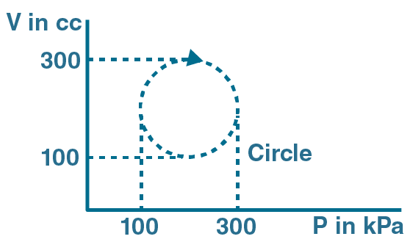Solution:

In the given case heat absorbed = Area of the circle

and, ΔQ = ΔW = area of circle

Diameter of the circle =300-100=200, so radius = 100

Area of circle = π × 100 × 100 × 10-6 × 103 = 31.4

[10-6 × 103 added because volume and pressure are given in cc and kPa respectively)

Thus, Heat absorbed by a system = 31.4 J.

Question 11: A gas is taken through a cyclic process ABCA as shown in the figure. If 2.4 cal of heat is given in the process, what is the value of J?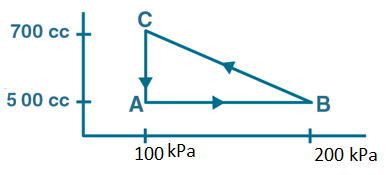Solution:

work done by the gas: ΔW = PΔV

Work done in path AB = 0 as VA=VB.

Work done in path CA = ΔW1 = PA(VA-VC) = 100 × 103× (500-700)×10-6 = -20J

Work done in path BC = ΔW2 = Pavg(VC – VB) …(1)

Pavg = [PB + PC]/2 = [100×103+200×103]/2 = 150 x 103 Pa

(1)=> ΔW2 = 150 x 103 (700-500)10-6 = 30 J

Total work done in process ABCA = ΔW = ΔW1 + ΔW2 =30 – 20 = 10 J

From first law of thermodynamics,

ΔQ = ΔU + ΔW

In cyclic process, ΔU = 0

=> ΔQ = ΔW

ΔQ = 2.4 cal (Given)

2.4 x J = 10 Joule

J = 10/2.4 = 4.17 Joule/cal

Question 12: A substance is taken through the process abc as shown in the figure. If the internal energy of the substance increases by 5000 J and heat of 2625 cal is given to the system, calculate the value of J.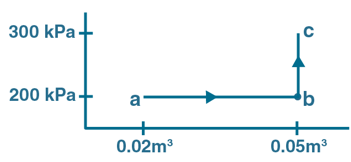Solution:

Work done in process abc = ΔW = Wab + Wbc

ΔW = Pa(Vb – Va) + 0

(As, Vb = Vc => Wbc = 0)

ΔW = 200 × 103 × (0.05-0.02) = 6000J

From first law of thermodynamics, ΔQ = ΔU + ΔW

Change in internal energy= ΔU = 5000J (Given)

=> ΔQ = 5000J + 6000J = 11000 J

But Heat given to system = 2625cal = 2625×J J (Given)

=> 2625×J = 11000

or J = 4.19 Joule/cal

Question 13: A gas is taken along the path AB as shown in the figure. If 70 cal of heat is extracted from the gas in the process, calculate the change in the internal energy of the system.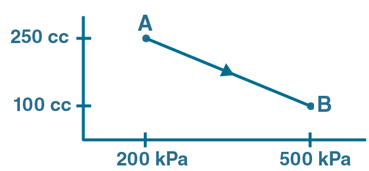Solution:

ΔQ = -70 cal = -70×4.2 = -294J (Given)

and, ΔW = PΔV

Where P = Pavg = [PA+PB]/2 = [200×103+500×103]/2 = 350×103 Pa

ΔW = 350 x 103 x (250 – 100) x 10-6

= -52.5 J

From first law of thermodynamics, ΔQ = ΔU + ΔW

=> ΔU = -294 – (-52.5) = -241.5 J

Question 14: The internal energy of a gas is given by U = 1.5 pV. It expands from 100 cm3 to 200 cm3 against a constant pressure of 1.0 × 105 Pa. Calculate the heat absorbed by the gas in the process.

Solution:

Change in volume ΔV = (200-100)×10-6 m3 = 10-4 m3

change in internal energy ΔU = 1.5 pΔV = 1.5×1.0×105×10-4 = 15J

work done by the gas: ΔW = pΔV = 1.0×105×10-4 = 10J

From first law of thermodynamics, ΔQ = ΔU + ΔW = 15 + 10 = 25 J

Question 15: A gas is enclosed in a cylindrical vessel fitted with a frictionless piston. The gas is slowly heated for some time. During the process, 10 J of heat is supplied, and the piston is found to move out 10 cm. Find the increase in the internal energy of the gas. The area of cross section of the cylinder = 4 cm2 and the atmospheric pressure = 100 kPa.

Solution:

Heat supplied to system =ΔQ = 10J

Atmospheric pressure =P= 100 kPa =100×103 Pa

Displacement of the piston d = 10 cm

Area of cross section of cylinder = A = 4 cm2

Now, ΔV = A x 10 cm3 = 4 x 10 cm3 = 40 x 10-6 m3

ΔW = P Δv = 100 x 103 x 40 x 10-6 = 4 cm3

Now, 10 = ΔU +ΔW

=> ΔU = 6J

Question 16: A gas is initially at a pressure of 100 kPa and its volume is 2.0 m3. Its pressure is kept constant and the volume is changed from 2.0 m3 to 2.5 m3. Its volume is now kept constant and the pressure is increased from 100 kPa to 200 kPa. The gas is brought back to its initial state, the pressure varying linearly with its volume.

(a) Whether the heat is supplied to or extracted from the gas in the complete cycle?

(b) How much heat was supplied or extracted?

Solution:

From graph

Va = 2m3 ; Vb = Vc = 2.5m3 ; Pa = Pb = 100kPa = 100 x 103 Pa

Pc = 200 kPa = 200x 103 Pa

Work done in process ABCA = area enclosed by the triangle ABC

=> ΔW = 0.5 × BC × AB

= 0.5 ×(200-100)× 103 x (2.5-2)

= 25000 J

(a) From first law of thermodynamics: ΔQ = ΔU + ΔW

Here ΔU = 0, as Process ABCA is a cyclic process => change in internal energy will be zero.

=> ΔQ = ΔW =25000 J

the heat is extracted from the system.

(b) Amount of heat supplied = work done by the gas in the cyclic process

i.e. ΔQ = ΔW = 25000J.

Question 17: Consider the cyclic process ABCA, shown in the figure, performed on a sample 2.0 mol of an ideal gas. A total of 1200 J of heat is withdrawn from the sample in the process. Find the work done by the gas during the part BC.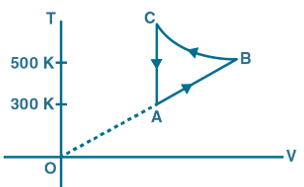Solution:

Heat extracted from the system = ΔQ = -1200J (given)

Number of moles of the gas = n=2.0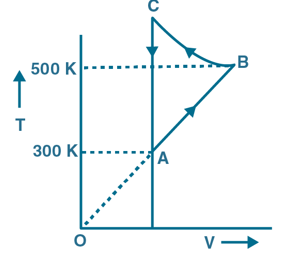We know, ΔW = P ΔV

Since VA = VC => work done along line CA will be zero.

Total work done = ΔW = WAB + WBC = P(VB-VA) + WBC

But we know that ideal gas equation, PV = nRT

Therefore, PΔV=nRΔT

=> ΔW = nR(TB – TA) + WBC …(1)

From first law of thermodynamics, ΔQ = ΔU + ΔW

Since Process ABCA is a cyclic process, ΔU = 0

=> ΔQ = ΔW = nR(TB – TA) + WBC

[Using (1)]

-1200 = 2x 8.31(500 – 300) + WBC

=> WBC = -4524 J

Question 18: Figure shows the variation in the internal energy U with the volume V of 2.0 mol of an ideal gas in a cyclic process abcda. The temperatures of the gas at b and c are 500 K and 300 K respectively. Calculate the heat absorbed by the gas during the process.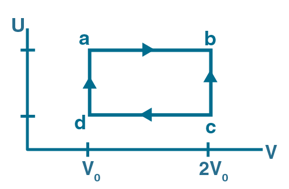Solution:

Here n = 2 moles

From figure, ΔV = 0 for path bc and da.

Hence ΔQ = ΔW

We know that, work done in an isothermal process: W = nRT ln[Vf/Vi]

=> ΔW = ΔWab + ΔWcd

= nRTa ln [Vb/Va] + nRTc ln [Vd/Vc]

= 2 x 8.31 x 500 x ln(2V0/V0) + 2 x 8.31 x 300 x ln(V0/2V0)

= 8310 x ln(2) – 4986 x ln(2)

= 2304.02 J

Question 19: Find the change in the internal energy of 2 kg of water as it is heated from 0°C to 4°C. The specific heat capacity of water is 4200 J kg–1 K–1 and its densities at 0°C and 4°C are 999.9 kg m–3 and 1000 kg m–3 respectively. Atmospheric pressure = 105 Pa.

Solution:

m = 2kg, ΔT = 4℃ -0℃ =4℃

c = 4200 J kg–1K–1

Density of water at 0°C = 999.9 kg m–3

Density of water at 4°C=1000 kg m–3

We know that specific heat capacity: c = ΔQ/mΔT

=> ΔQ = cm ΔT

= 4200 x 2 x 4 = 33600J

Volume at 0oC:

V1 = m/[density at 0oC] = 2/999.9 m3

Volume at 4oC:

V2 = m/[density at 4oC] = 2/1000 m3

Work done by the gas: ΔW = PΔV = P(V2 – V1)

= 105 x (2/1000 – 2/999.9)

= -0.02 J

From first law of thermodynamics: ΔU = ΔQ – ΔW

= 33600 – (-0.02)

= 33599.98J

change in internal energy is 33599.98J.

Question 20: Calculate the increase in the internal energy of 10g of water when it is heated from 0°C to 100°C and converted into steam at 100 kPa. The density of steam = 0.6 kg m–3. The specific heat capacity of water = 4200 J kg–1 °C–1 and the latent heat of vaporization of water = 2.25 × 106 J kg–1.

Solution:

Pressure = P = 100kPa = 100×105 Pa

Change in temperature = ΔT = (100-0) oC = 100oC

Density of water ρ = 1000 kg m-3

specific heat capacity: c = ΔQ/mΔT

=> ΔQ = cm ΔT

In this question,

=> ΔQ = mL + cmΔT …(1)

Where,

Mass of water m=10g =0.010kg

latent heat of vaporization of water= L = 2.25 × 106 J kg–1.

c = 4200 J kg–1 °C–1

Putting all the values in (1), we get

ΔQ = 26700J

Now, work done by the gas: ΔW=PΔV

here ΔV = (m/ρ’ – m/ρ)

=> ΔW = 105 x [0.01/0.06 – 0.01/1000]

= 1699J

By first law of thermodynamics, ΔQ = ΔU + ΔW

= 26700 – 1699

=> ΔQ = 25001J

Question 21: Figure shows a cylindrical tube of volume V with adiabatic walls containing an ideal gas. The internal energy of this ideal gas is given by 1.5 nRT. The tube is divided into two equal parts by a fixed diathermic wall. Initially, the pressure and the temperature are p1, T1 on the left and p2, T2 on the right. The system is left for sufficient time so that the temperature becomes equal on the two sides.

(a) How much work has been done by the gas on the left part?

(b) Find the final pressures on the two sides.

(c) Find the final equilibrium temperature.

(d) How much heat has flown from the gas on the right to the gas on the left?Solution:

(a) Since all wall can not be moved thus ΔU = 0 and ΔQ = 0

=> ΔW = 0

(b) Applying ideal gas equation in the left chamber before and after equilibrium

P1V/2 = nRT1 and (P1‘ V)/2 = nRT

=> P1/T1 = P1‘ V/T

=> P1‘ = P1T/T1

using value of T (Solved in part (c))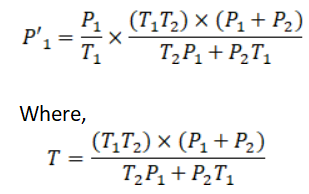Similarly, P’2 is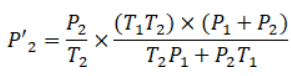(c) Let final pressure in LHS and RHS be P1 and P2

=> n1 = P1V/2RT1 and n2 = P2V/2RT2

As, Total number of moles n=n1 +n2

n = P1V/2RT1 + n2 = P2V/2RT2

n = V/2R [(T2P1 + P2T1)/(T1T2)] …(1)

The internal energy of ideal gas : U = nCv T

Where Cv = molar specific heat at constant volume

According to question, U = 1.5nRT

=> nCv T = 1.5 nRT

=> Cv = 1.5R

Internal energy of the left chamber = U1 = n1CvT1

Internal energy of right chamber = U2 = n2CvT2

Total internal energy U = U1 + U2

(1.5)nRT= n1CvT2 + n2CvT2

(1.5)nRT = 1.5R(n1T1 + n2T2)

nT = n1T1 + n2T2

substituting the value of n, n1 and n2, we get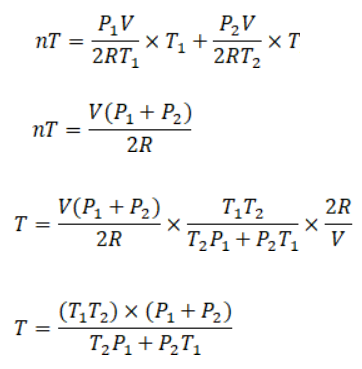which is the final equilibrium temperature.

(d) The internal energy of ideal gas: U = nCvT

From part (a), ΔW = 0 for the right chamber of the tube.

=> ΔQ = ΔU …(a)

Change in internal energy of the right chamber after equilibrium will be

ΔU = n2cvT2 – n2cvT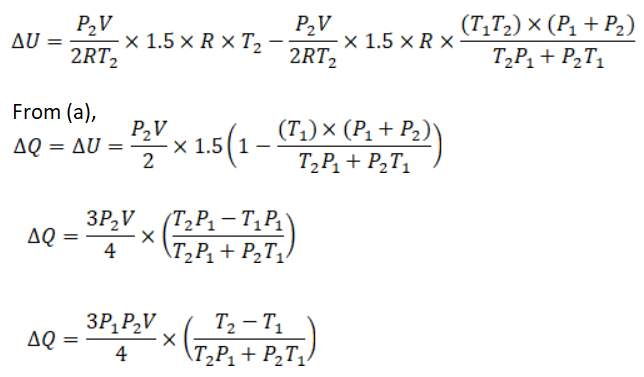Which is the heat flown from left to right chamber.

Question 22: An adiabatic vessel of total volume V is divided into two equal parts by a conducting separator. The separator is fixed in this position. The part on the left contains one mole of an ideal gas (U = 1.5 nRT) and the part on the right contains two moles of the same gas. Initially, the pressure on each side is p. The system is left for sufficient time so that a steady state is reached. Find

(a) the work done by the gas in the left part during the process.

(b) the temperature on the two sides in the beginning,

(c) the final common temperature reached by the gases,

(d) the heat given to the gas in the right part and

(e) the increase in the internal energy of the gas in the left part.

Solution: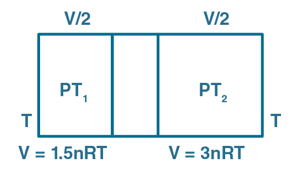(a) As the conducting wall is fixed the work done by the gas on the left part during the process is zero. Because change in volume will be zero due to fixed position of the wall.

(b)For left side:

Let p = initial pressure on both sides of the wall.

T1 = initial temperature

number of moles = 1

Also, volume = V/2

We know, PV = nRT

In this situation, above equation can be written as

PV/2 = nRT1

=> T2 = PV/2R

Similarly, for the right part

number of moles = 2

=> T2 = PV/4R

(c) The internal energy of the gas: U = 1.5 nRT

and internal energy of ideal gas: U = n Cv T

Total moles = n = n1 + n2 = 1 + 2 = 3

=> U = 3 Cv T

Let U1 and U2 be the internal energy of the left and right part respectively.

So, U1 = n1 Cv T1 = CvT1 and U2 = n2 Cv T2 = 2 Cv T2

Here, Cv will be same since gas is same on both the parts

=> U = U1 + U2

=> 3 Cv T = CvT1 + 2 Cv T2

=> 3T = T1 + 2T2

Using values of T1 and T2

=>T = pV/3R

(d) As per part (a), ΔW=0 for the right part of the vessel.

We know, ΔQ=ΔU+ΔW

=> ΔQ = ΔU

U = 1.5 nRT (Given)

Heat given to the right part = ΔU = 1.5 n R ΔT

For the right part, change in internal energy after equilibrium has reached will be due to the change in temperature from T2 to T.

ΔU = 1.5 n2 R (T – T2)

Substituting Values of n2, T1 and T2, we get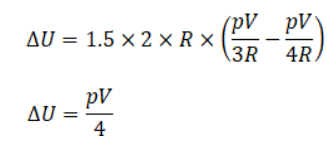(e) From part (a), Since ΔW is zero as the volume is fixed.

Here, ΔQ = -ΔU (left part)

=> ΔU = -PV/4